Product Sales Analysis Sales Potential vs Sales Growth

• Slides: 13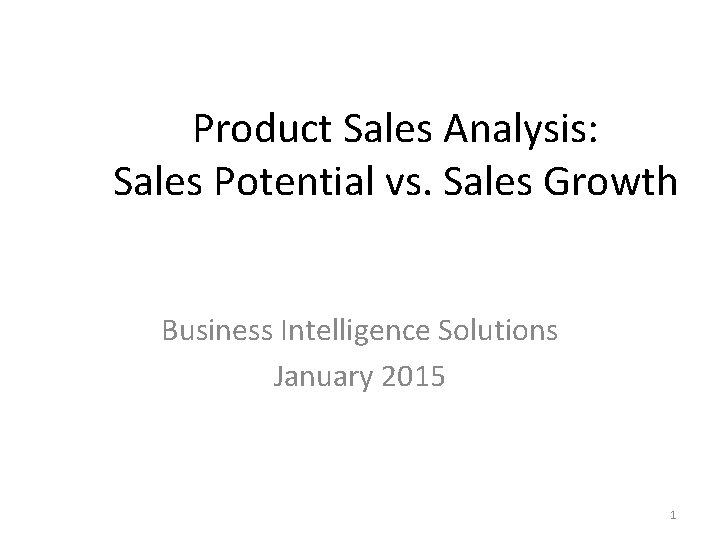Product Sales Analysis: Sales Potential vs. Sales Growth Business Intelligence Solutions January 2015 1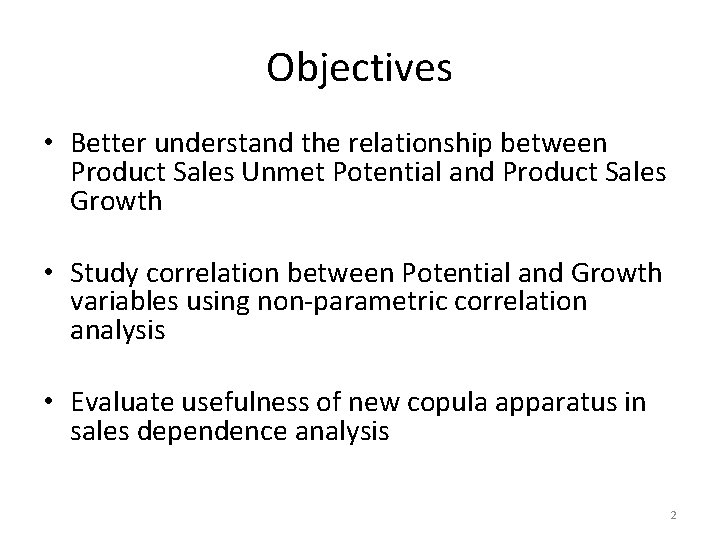Objectives • Better understand the relationship between Product Sales Unmet Potential and Product Sales Growth • Study correlation between Potential and Growth variables using non-parametric correlation analysis • Evaluate usefulness of new copula apparatus in sales dependence analysis 2Product Sales Unmet Potential vs. Product Sales Growth (360 observations data set) Correlation = - 0. 14 P-value = 0. 006 Product Unit Growth Product Dollars Growth Correlation = - 0. 35 P-value < 0. 0001 Product Unit Unmet Potential Product Dollars Unmet Potential 3Relationship between Sales Growth and Sales Unmet Potential variables • Correlations between Product Sales Growth and Product Sales Unmet Potential variables are highly significant and negative • On average, the larger the growth of sales, the smaller sales unmet potential • The highest negative correlation (-0. 35 ) is between Product Dollar Growth and Product Dollar Unmet Potential 4Product Unit Unmet Potential Distribution Histogram and Best fit distribution Cumulative empirical and best fit distribution Mean = 589 units Median = 523 units Dagum distribution - best fit out of 15 candidates Blue – actual data Red – fitted Dagum distribution 5Product Unit Growth Distribution Histogram and Best fit distribution Cumulative empirical and best fit distribution Mean = - 836 units Median = - 550 units Burr distribution - best fit out of 5 candidates Blue – actual data Red – fitted Burr distribution 6Empirical Copula for 2 -dimensional Product Potential – Growth Unit data Fitted Copula (generated 360 observations) Product Unit Growth percentiles Data (original 360 observations) Product Unit Unmet Potential percentiles Note: Product Unit Unmet Potential percentiles are calculated using fitted Dagum distribution, and Product Unit Growth percentiles are calculated using fitted Burr distribution 7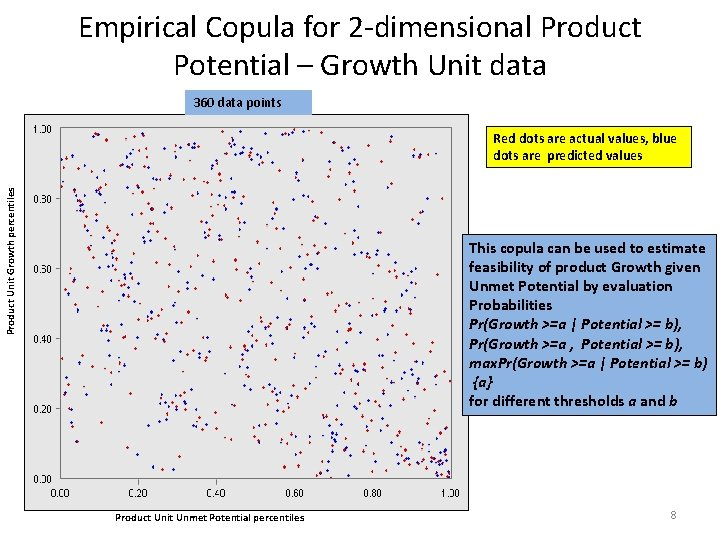Empirical Copula for 2 -dimensional Product Potential – Growth Unit data 360 data points Product Unit Growth percentiles Red dots are actual values, blue dots are predicted values This copula can be used to estimate feasibility of product Growth given Unmet Potential by evaluation Probabilities Pr(Growth >=a | Potential >= b), Pr(Growth >=a , Potential >= b), max. Pr(Growth >=a | Potential >= b) {a} for different thresholds a and b Product Unit Unmet Potential percentiles 8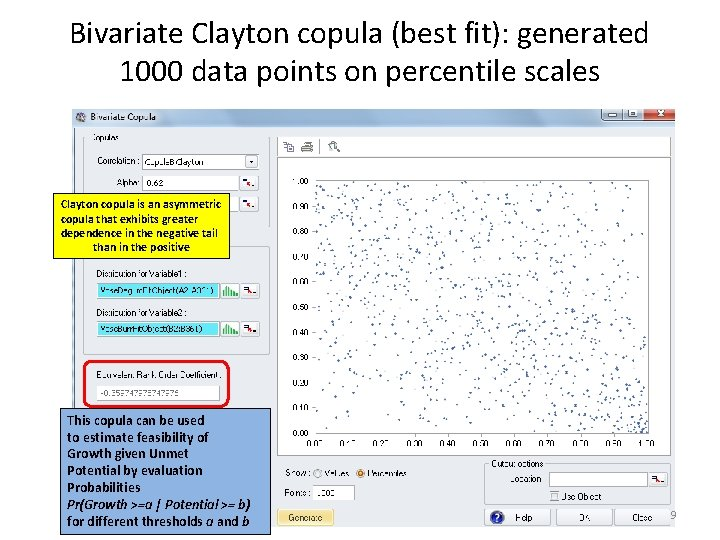Bivariate Clayton copula (best fit): generated 1000 data points on percentile scales Clayton copula is an asymmetric copula that exhibits greater dependence in the negative tail than in the positive This copula can be used to estimate feasibility of Growth given Unmet Potential by evaluation Probabilities Pr(Growth >=a | Potential >= b) for different thresholds a and b 9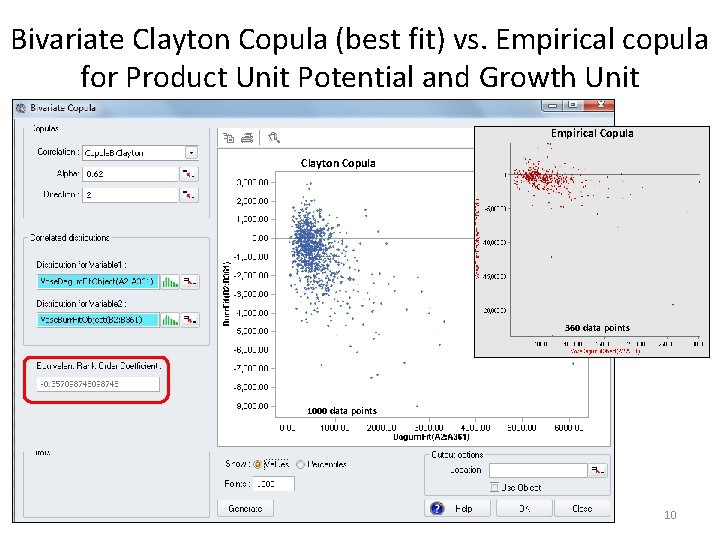Bivariate Clayton Copula (best fit) vs. Empirical copula for Product Unit Potential and Growth Unit Empirical Copula Clayton Copula 360 data points 1000 data points 10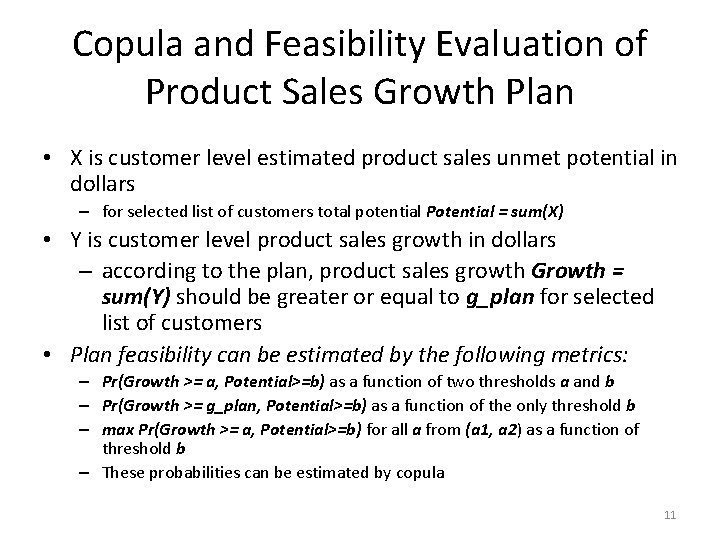Copula and Feasibility Evaluation of Product Sales Growth Plan • X is customer level estimated product sales unmet potential in dollars – for selected list of customers total potential Potential = sum(X) • Y is customer level product sales growth in dollars – according to the plan, product sales growth Growth = sum(Y) should be greater or equal to g_plan for selected list of customers • Plan feasibility can be estimated by the following metrics: – Pr(Growth >= a, Potential>=b) as a function of two thresholds a and b – Pr(Growth >= g_plan, Potential>=b) as a function of the only threshold b – max Pr(Growth >= a, Potential>=b) for all a from (a 1, a 2) as a function of threshold b – These probabilities can be estimated by copula 11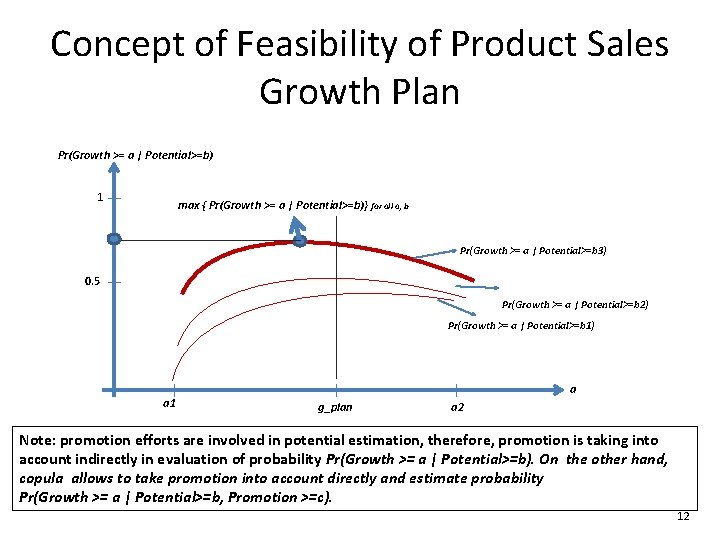Concept of Feasibility of Product Sales Growth Plan Pr(Growth >= a | Potential>=b) 1 max { Pr(Growth >= a | Potential>=b)} for all a, b Pr(Growth >= a | Potential>=b 3) 0. 5 Pr(Growth >= a | Potential>=b 2) Pr(Growth >= a | Potential>=b 1) a 1 a g_plan a 2 Note: promotion efforts are involved in potential estimation, therefore, promotion is taking into account indirectly in evaluation of probability Pr(Growth >= a | Potential>=b). On the other hand, copula allows to take promotion into account directly and estimate probability Pr(Growth >= a | Potential>=b, Promotion >=c). 12Summary • Product Sales Unmet Potential is not Product Sales Unmet Potential is an additional maximum value that can be generated by product sales during next year in principle if the promotion efforts and the mechanism of data generation will be the same • All three non-parametric measures of association (Spearman, Kendall, and Hoeffding) are indicative of existing strong correlation between Product Unmet Potential and Product Growth variables • Product Unit Unmet Potential is negatively correlated with Product Sales Growth: – - 0. 35 for Unit Growth – - 0. 14 for Dollars Growth with p-value < 0. 001 (Spearman correlation) • Copula is useful approach to estimate dependence structure in multidimensional settings, especially tail dependence, and evaluate probability of any event, and in particular, feasibility of a planned product growth 13